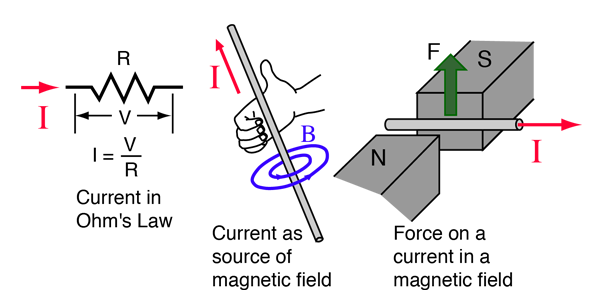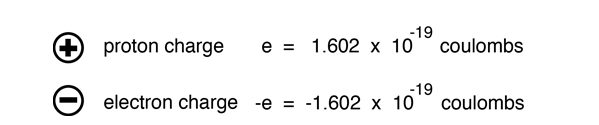# Electric Current

Electric current is the rate of charge flow past a given point in an electric circuit, measured in Coulombs/second which is named Amperes. In most DC electric circuits, it can be assumed that the resistance to current flow is a constant so that the current in the circuit is related to voltage and resistance by Ohm's law. The standard abbreviations for the units are 1A = 1C/s.Microscopic view of electric current
 Measurement with ammeter
 Conventional electric current direction
Index

Electric Circuits

 HyperPhysics***** Electricity and Magnetism R Nave
Go Back

# Electric Charge

The unit of electric charge is the Coulomb (abbreviated C). Ordinary matter is made up of atoms which have positively charged nuclei and negatively charged electrons surrounding them. Charge is quantized as a multiple of the electron or proton charge:The influence of charges is characterized in terms of the forces between them (Coulomb's law) and the electric field and voltage produced by them. One Coulomb of charge is the charge which would flow through a 120 watt lightbulb (120 volts AC) in one second. Two charges of one Coulomb each separated by a meter would repel each other with a force of about a million tons!

The rate of flow of electric charge is called electric current and is measured in Amperes.

In introducing one of the fundamental properties of matter, it is perhaps appropriate to point out that we use simplified sketches and constructs to introduce concepts, and there is inevitably much more to the story. No significance should be attached to the circles representing the proton and electron, in the sense of implying a relative size, or even that they are hard sphere objects, although that's a useful first construct. The most important opening idea, electrically, is that they have a property called "charge" which is the same size, but opposite in polarity for the proton and electron. The proton has 1836 times the mass of the electron, but exactly the same size charge, only positive rather than negative. Even the terms "positive" and "negative" are arbitrary, but well-entrenched historical labels. The essential implication of that is that the proton and electron will strongly attract each other, the historical archtype of the cliche "opposites attract". Two protons or two electrons would strongly repel each other. Once you have established those basic ideas about electricity, "like charges repel and unlike charges attract", then you have the foundation for electricity and can build from there.

From the precise electrical neutrality of bulk matter as well as from detailed microscopic experiments, we know that the proton and electron have the same magnitude of charge. All charges observed in nature are multiples of these fundamental charges. Although the standard model of the proton depicts it as being made up of fractionally charged particles called quarks, those fractional charges are not observed in isolation -- always in combinations which produce +/- the electron charge.

An isolated single charge can be called an "electric monopole". Equal positive and negative charges placed close to each other constitute an electric dipole. Two oppositely directed dipoles close to each other are called an electric quadrupole. You can continue this process to any number of poles, but dipoles and quadrupoles are mentioned here because they find significant application in physical phenomena.

One of the fundamental symmetries of nature is the conservation of electric charge. No known physical process produces a net change in electric charge.

Index

Coulomb's Law

Electromagnetic force

 HyperPhysics***** Electricity and Magnetism R Nave
Go Back

# Conventional Electric Current

Although it is electrons which are the mobile charge carriers which are responsible for electric current in conductors such as wires, it has long been the convention to take the direction of electric current as if it were the positive charges which are moving. Some texts reverse this convention and take electric current direction as the direction the electrons move, an obviously more physically realistic direction, but the vast majority of references use the conventional current direction and that convention will be followed in most of this material. In common applications such as determining the direction of force on a current carrying wire, treating current as positive charge motion or negative charge motion gives identical results. Besides the advantage of agreeing in direction with most texts, the conventional current direction is the direction from high voltage to low voltage, high energy to low energy, and thus has some appeal in its parallel to the flow of water from high pressure to low (see water analogy).

Index

Electric Circuits

 HyperPhysics***** Electricity and Magnetism R Nave
Go Back